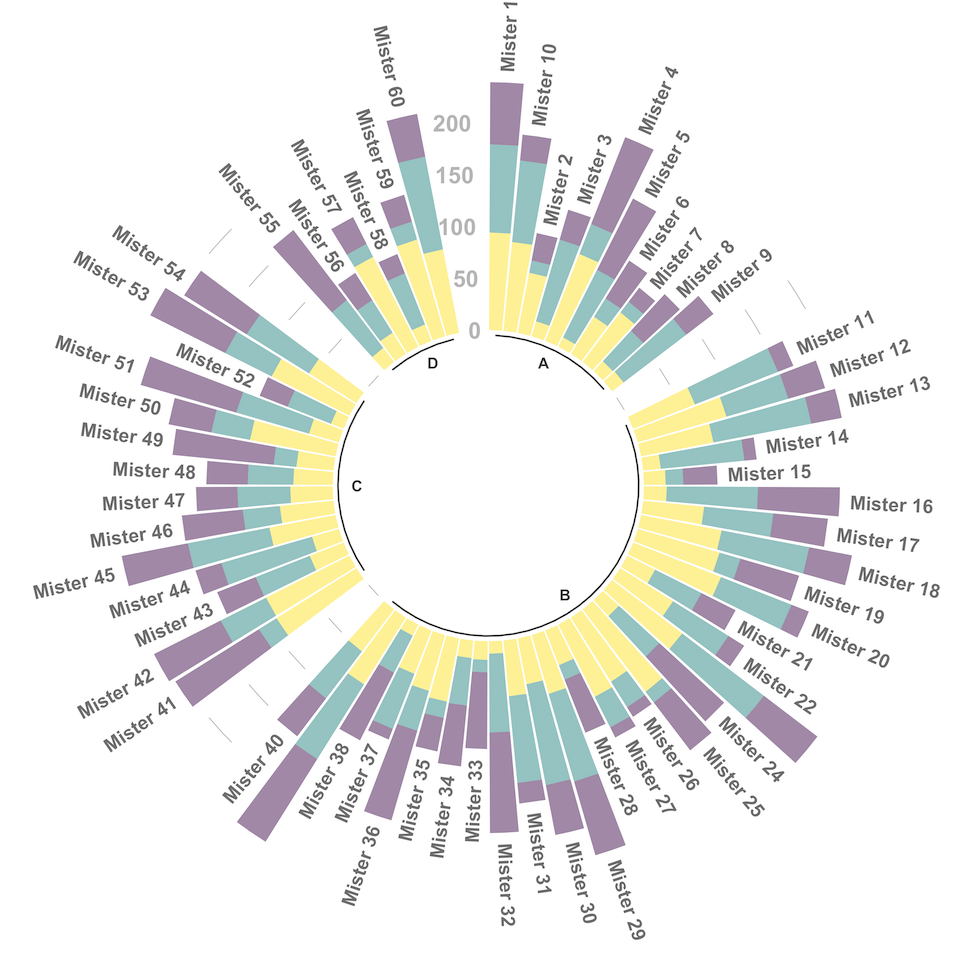# Circular stacked barplot

A circular barplot is a barplot where bars are displayed along a circle instead of a line. This page aims to teach you how to make a grouped and stacked circular barplot with `R` and `ggplot2`.A circular barplot is a barplot where bars are displayed along a circle instead of a line. This page aims to teach you how to make a grouped and stacked circular barplot. I highly recommend to visit graph #295, #296 and #297 Before diving into this code, which is a bit rough.

I tried to add as many comments as possible in the code, and thus hope that the method is understandable. If it is not, please comment and ask supplementary explanations.

You first need to understand how to make a stacked barplot with ggplot2. Then understand how to properly add labels, calculating the good angles, flipping them if necessary, and adjusting their position. The trickiest part is probably the one allowing to add space between each group. All these steps are described one by one in the circular barchart section.

``````# library
library(tidyverse)
library(viridis)

# Create dataset
data <- data.frame(
individual=paste( "Mister ", seq(1,60), sep=""),
group=c( rep('A', 10), rep('B', 30), rep('C', 14), rep('D', 6)) ,
value1=sample( seq(10,100), 60, replace=T),
value2=sample( seq(10,100), 60, replace=T),
value3=sample( seq(10,100), 60, replace=T)
)

# Transform data in a tidy format (long format)
data <- data %>% gather(key = "observation", value="value", -c(1,2))

# Set a number of 'empty bar' to add at the end of each group
empty_bar <- 2
nObsType <- nlevels(as.factor(data\$observation))
to_add <- data.frame( matrix(NA, empty_bar*nlevels(data\$group)*nObsType, ncol(data)) )
data <- data %>% arrange(group, individual)
data\$id <- rep( seq(1, nrow(data)/nObsType) , each=nObsType)

# Get the name and the y position of each label
label_data <- data %>% group_by(id, individual) %>% summarize(tot=sum(value))
number_of_bar <- nrow(label_data)
angle <- 90 - 360 * (label_data\$id-0.5) /number_of_bar     # I substract 0.5 because the letter must have the angle of the center of the bars. Not extreme right(1) or extreme left (0)
label_data\$hjust <- ifelse( angle < -90, 1, 0)
label_data\$angle <- ifelse(angle < -90, angle+180, angle)

# prepare a data frame for base lines
base_data <- data %>%
group_by(group) %>%
summarize(start=min(id), end=max(id) - empty_bar) %>%
rowwise() %>%
mutate(title=mean(c(start, end)))

# prepare a data frame for grid (scales)
grid_data <- base_data
grid_data\$end <- grid_data\$end[ c( nrow(grid_data), 1:nrow(grid_data)-1)] + 1
grid_data\$start <- grid_data\$start - 1
grid_data <- grid_data[-1,]

# Make the plot
p <- ggplot(data) +

geom_bar(aes(x=as.factor(id), y=value, fill=observation), stat="identity", alpha=0.5) +
scale_fill_viridis(discrete=TRUE) +

# Add a val=100/75/50/25 lines. I do it at the beginning to make sur barplots are OVER it.
geom_segment(data=grid_data, aes(x = end, y = 0, xend = start, yend = 0), colour = "grey", alpha=1, size=0.3 , inherit.aes = FALSE ) +
geom_segment(data=grid_data, aes(x = end, y = 50, xend = start, yend = 50), colour = "grey", alpha=1, size=0.3 , inherit.aes = FALSE ) +
geom_segment(data=grid_data, aes(x = end, y = 100, xend = start, yend = 100), colour = "grey", alpha=1, size=0.3 , inherit.aes = FALSE ) +
geom_segment(data=grid_data, aes(x = end, y = 150, xend = start, yend = 150), colour = "grey", alpha=1, size=0.3 , inherit.aes = FALSE ) +
geom_segment(data=grid_data, aes(x = end, y = 200, xend = start, yend = 200), colour = "grey", alpha=1, size=0.3 , inherit.aes = FALSE ) +

# Add text showing the value of each 100/75/50/25 lines
ggplot2::annotate("text", x = rep(max(data\$id),5), y = c(0, 50, 100, 150, 200), label = c("0", "50", "100", "150", "200") , color="grey", size=6 , angle=0, fontface="bold", hjust=1) +

ylim(-150,max(label_data\$tot, na.rm=T)) +
theme_minimal() +
theme(
legend.position = "none",
axis.text = element_blank(),
axis.title = element_blank(),
panel.grid = element_blank(),
plot.margin = unit(rep(-1,4), "cm")
) +
coord_polar() +

# Add labels on top of each bar
geom_text(data=label_data, aes(x=id, y=tot+10, label=individual, hjust=hjust), color="black", fontface="bold",alpha=0.6, size=5, angle= label_data\$angle, inherit.aes = FALSE ) +

geom_segment(data=base_data, aes(x = start, y = -5, xend = end, yend = -5), colour = "black", alpha=0.8, size=0.6 , inherit.aes = FALSE )  +
geom_text(data=base_data, aes(x = title, y = -18, label=group), hjust=c(1,1,0,0), colour = "black", alpha=0.8, size=4, fontface="bold", inherit.aes = FALSE)

# Save at png
ggsave(p, file="output.png", width=10, height=10)``````

Related chart types

## Contact

This document is a work by Yan Holtz. Any feedback is highly encouraged. You can fill an issue on Github, drop me a message on Twitter, or send an email pasting yan.holtz.data with gmail.com.